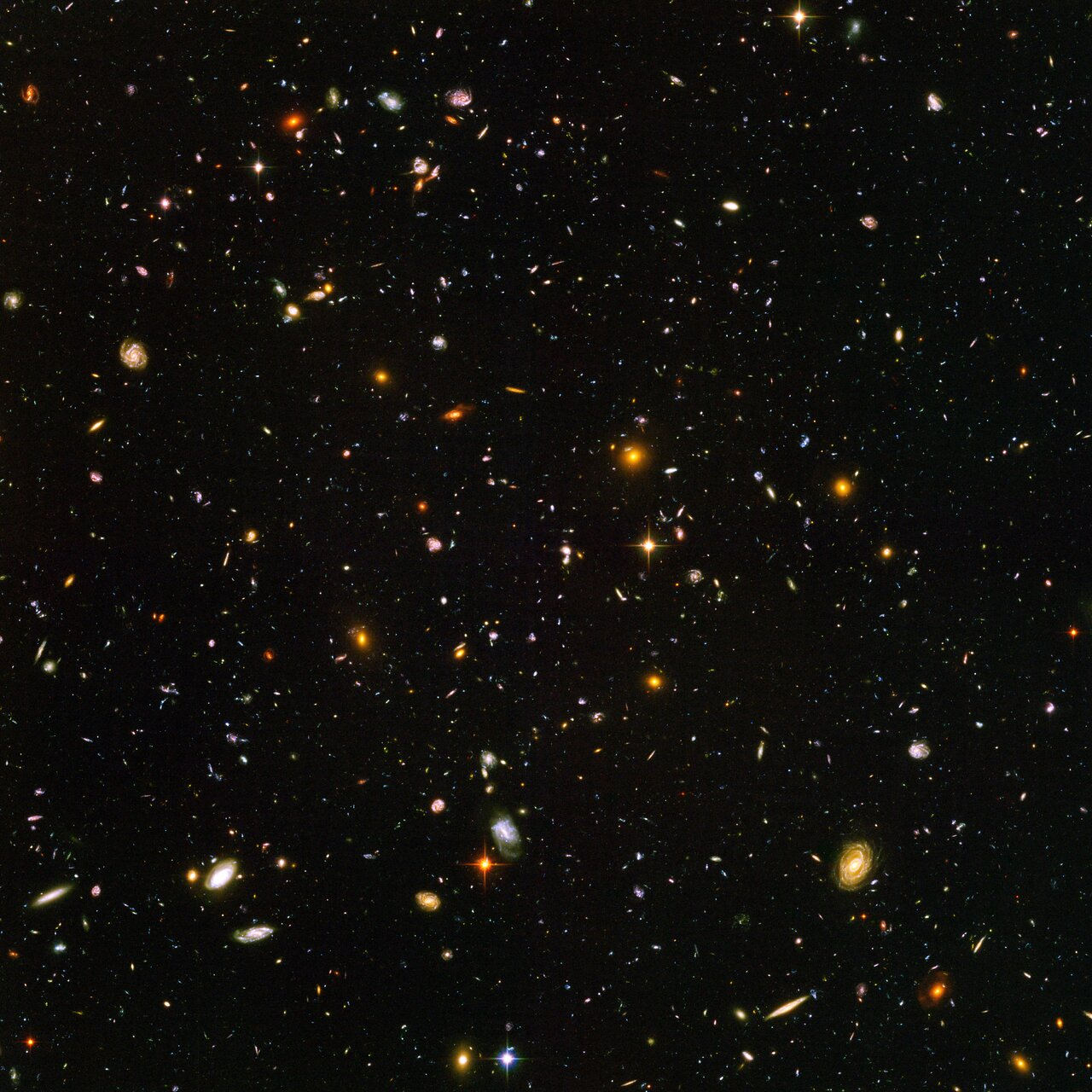# Is the universe infinite?

I have been returning to this question lately – and I see three possible answers:

1. The universe is finite
2. The universe is infinite
3. The universe is “infinitely finite”Option 1 introduces an “edge problem” where the particles at the end of the universe will have interacting forces on only one side. If this option is true, the universe started out as point-like Big Bang, satisfying the requirements for a Black Hole.

If Option 2 is true, the universe has always been infinite since nothing can go from finite to infinite (or vice versa). It started out as infinitely large and very dense at the Big Bang, satisfying the requirements for a Black Hole at all areas of space.

Option 3 would be similar to moving on Earth’s surface – if you move straight in one direction, you eventually circle the Earth and end up where you started. The universe could be a 3 dimensional space residing in a higher dimensional space – if you travel in one direction, you would never reach an edge. Instead you can end up back where you started (given that the higher dimensional space is a uniform “sphere”). The universe could have started out as a small 4D+ space.

I can’t for the moment see other options. Please pitch in with your own views.

One question that often pop up with an infinite universe is this: “If the universe is infinite, would everything that can happen be bound to happen – and an infinitely amount of times?”. The usual answer when you Google this is “Yes.” The answer is the same for “If you throw a dice an infinite number of times, must you eventually roll a six? Must you in fact roll an infinite number of sixes?”

While it may be intuitively correct to answer “yes” to these questions, the answer is in fact wrong. Here’s why:

Consider the natural numbers 1, 2, 3, …

There are infinitely many of them … so 2 must show up more than once, right? Manifestly wrong.

But say we are talking about states of matter in a finite region. This would be modeled by using finitely many numbers, 1, 2, 3, say, and making an infinite list.

1, 2, 3, 1, 3, 1, 3, 1, 3, 1, 3, …

You say 2 must appear again … but it doesn’t. If you have finitely many states and infinitely many trials, all you can say for sure is that at least one state must reappear infinitely many times. But any particular state, such as the state that defines “you” or a pink elephant or a galaxy; might appear zero, one, 47, or infinitely many times.

It’s amazing how many otherwise smart people are fooled into thinking that “in an infinite universe, everything must happen.” This is manifestly false.

So even in an infinite universe, a chance of something specific happening is undecided. This is related to the equation$\frac{\infty}{\infty}$

which is mathematically undecided.

The question of whether the universe is finite, infinite or something else poses some interesting questions. And perhaps some interesting answers may arise.

# Infinity…

Some quick questions up for discussion:

• Is infinity pluss one the same as infinity?
• Is infinity times two the same as infinity?
• What is infinity minus infinity?
• What is infinity divided by infinity?
• Is the reciprocal of infinity equal to zero?
• Can something infinite have a beginning?